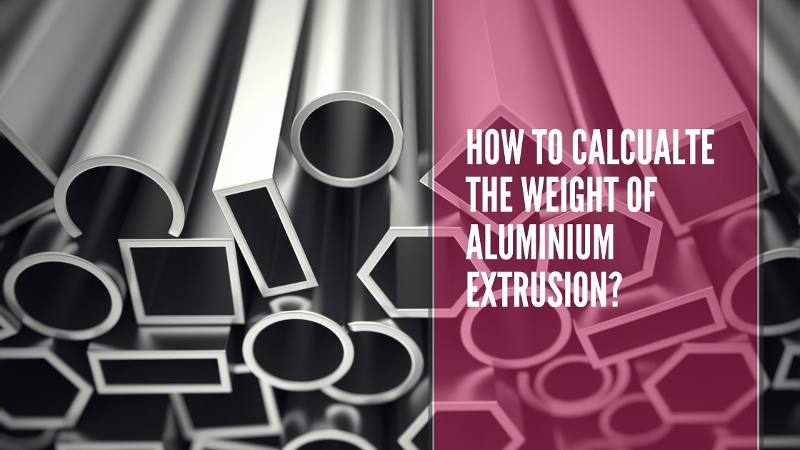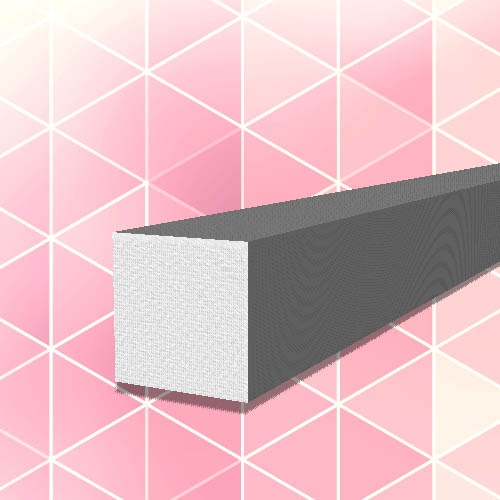# How to Calculate the Weight of Aluminium Extrusion?How do you work out how heavy an aluminium extrusion is?

The magic number you need is the theoretical weight per meter. Usually expressed in kilos per metre (kg/m). Once you have this you can work out the weight of any length of the profile in question.

The basic formula for working out the weight per meter of an aluminium extrusion is:

Cross sectional area (mm2) x Density (kg/cm3) = Theoretical weight per meter (kg/m)

But where do these numbers come from? Let's break it down into 3 easy steps...

#### Working out Cross Sectional Area (CSA)Cross sectional area is simply the area of a slice of your profile. If you can hark back to your time at school, it's just the ordinary area calculation i.e. length x width (or at least for a simple shape like a square).

It's important you use millimeters to work this out if you are going to follow all the steps in this guide.

So imagine a simple square bar like the one on the right. If it had a height of 30mm and a width of 30mm it would just be a simple calculation of:

30mm x 30mm = 900mm2

That's the easy part, this is just a square bar after all for more complicated shapes working out the CSA can be tricky.

If your profile is made up of lots of smaller squares, rectangles and triangles you could work out the area of each and then add them up to get the Cross Sectional Area.

If your shape is more complicated you will probably need to use Computer Aided Design (CAD) software to calculate the area for you. Most packages have simple tools that allow you to retrieve this number. If you are unsure you could always send a sketch to our in house product designer and we could draw it up and work it out for you.

#### Alloy Density

This is the easy part, simply take the density of the alloy from the list below. If you can't find the alloy you are looking for or are not sure which to pick try our aluminium alloy page for more information.

For a huge range of commercial applications such decorative trims, furniture, mechanical engineering, construction, windows, outdoor structures, etc. the most common alloys are 6060 and 6063.

In our square bar example in 6063 alloy the weight per metre would be:

900mm2 x 0.0027kg/cm3 = 2.43 kg/m

N.B. If you research densities on other websites you will usually find them listed in g/cm cubed, to make our calculation simple we have used kg/cm cubed. All you need to do is divide the number i.e. 2.7 for 6063 by 1000 to give you 0.0027.

 Alloy Typical end uses Density (kg/cm3) 2024 High strength aerospace 0.00278 3003 Formed heat exchangers 0.00273 5083 Marine use 0.00266 6060 General commercial use 0.00270 6061 High/medium strength commercial use 0.00270 6063 General commercial use 0.00270 6063A Consistent forming 0.00270 6082 High strength commercial use 0.00270 6463 High polish level commercial use 0.00269 7075 Highly stressed structural parts 0.00281

Data Source: Aluminium Association - International Alloy Designations (p12-22) many hundreds of alloys full chemical compositions and properties can be found in this handy document.

#### To wrap everything up

Now you have everything you need to work out the weight per metre. To find out the total weight per unit of your aluminium extrusion simply multiply the kg/m by the length of the finished product.

i.e. for our square bar, if I ordered a 6.3 metre length the weight of the unit would be:

2.43kg/m x 6.3m = 15.309 kg